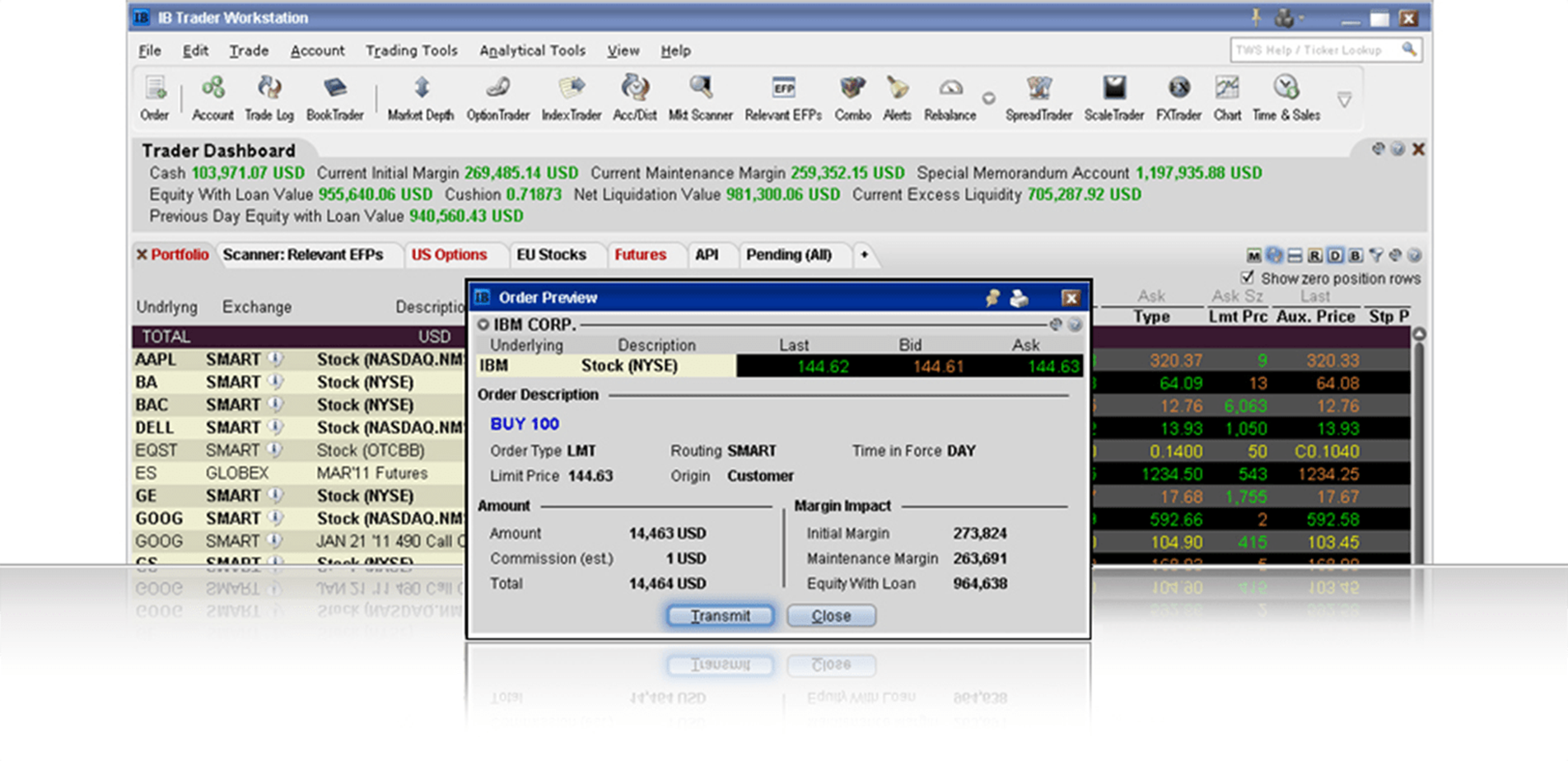# Model Navigator

Modify pricing assumptions and include them in the model price calculation using this sophisticated option model pricing tool.Part of our Price/Risk analytics suite, the Model Navigator allows you to modify the pricing assumptions used to calculate option model prices and recalculate the model price.

The Model Navigator is a sophisticated option model calculation engine that offers the following benefits to options traders:

• Easily view an option's structure
• Edit interest rates, dividends, lending yield and
implied volatilities
• Adjust the underlying yield so that the stock
lending rate can be reflected in the model
calculation
• Model prices display in a range of colors, letting
you quickly see where they fall in relation to the